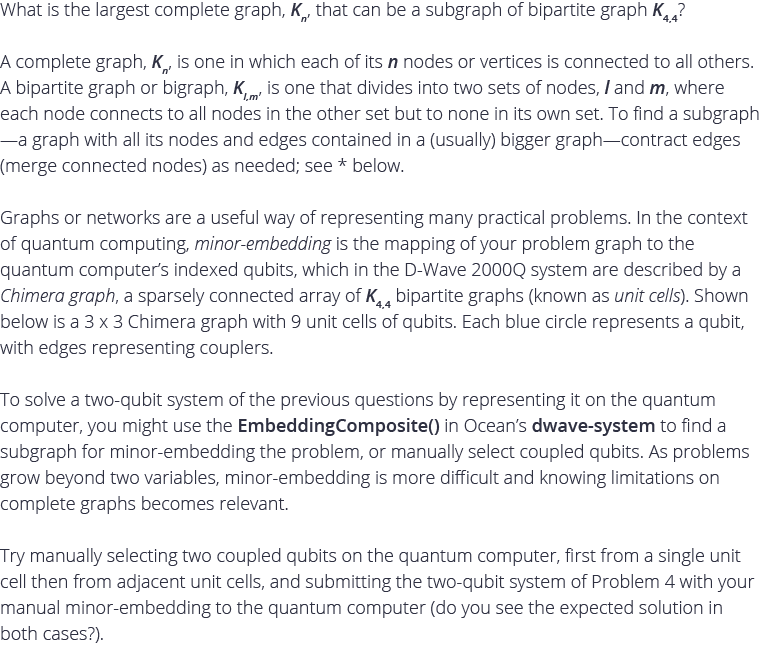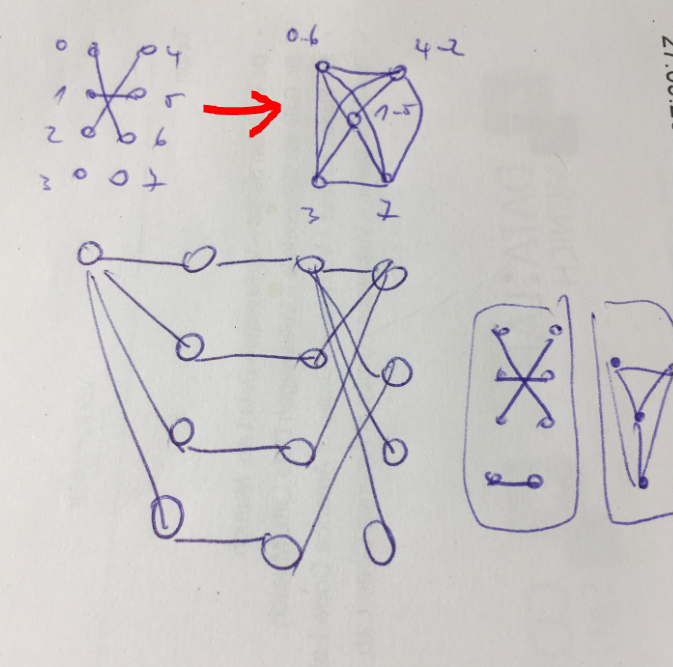# Problem 6: Find the Largest Complete Graph for a Chimera Unit Cell* Example AND Gate explains how nodes are merged (two or more qubits are chained to represent a single variable). Its code demonstrating minor-embedding a 3-variable problem on the quantum computer, both automatically and manually, is also helpful for this question.

** Ocean's dwave-system documentation is here.• The largest complete graph would be K5, that can be sub-graph of bipartite graph K4,4. See the first part of the code.

In part two, the problem in challenge 4 was manually embedded to qubit 5 and 13, which are in separate unit cells. Results look the same.

Below are the results screen and the code.

********** Part 1: Largest complete graph Kn that can be a sub-graph of bipartite graph K4,4 ***********

Assignment :
{'q1': {0}, 'q2': {4}, 'q3': {2, 6}, 'q4': {3, 5}, 'q5': {1, 7}}
Neighbourhood check :
{'q1': {'q5', 'q4', 'q2', 'q3'}, 'q2': {'q5', 'q1', 'q4', 'q3'}, 'q3': {'q4', 'q1', 'q2', 'q5'}, 'q4': {'q5', 'q1', 'q2', 'q3'}, 'q5': {'q4', 'q1', 'q2', 'q3'}}

********** Part 2: Problem 4 using manual embedding to qubit 5 and 13 (in two different unit cells)

|h0 = 0 |h1 = 1 | Energy = -0.200000 | Probability = 32.000000 %
|h0 = 1 |h1 = 0 | Energy = -0.200000 | Probability = 68.000000 %

• using entanglement effect (couplers), you can merge 2 physical qbits into 1 logical, gaining connectivity to another qbits, paying the price with consuming available physical qbits.

that way (using 3 couplers) you get from K4,4 bipartite grap, one (max) K5 complete subgraph.using more couplers you get less vertices.• Thanks to those of you who tried this problem!  Kudos to Yasas and Branislav for finding the answer we were looking for, which is K5.

Next, take a look at Problem 7, posted today.